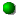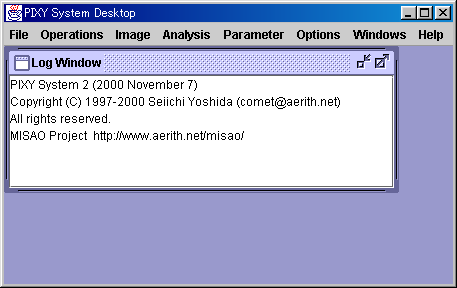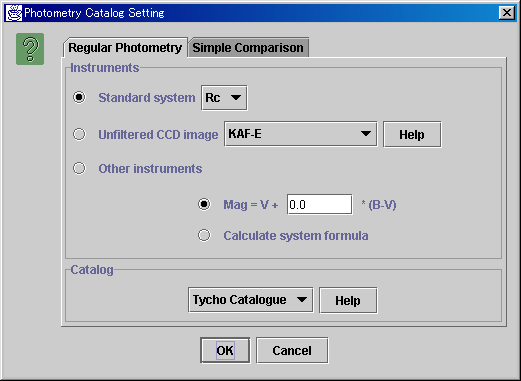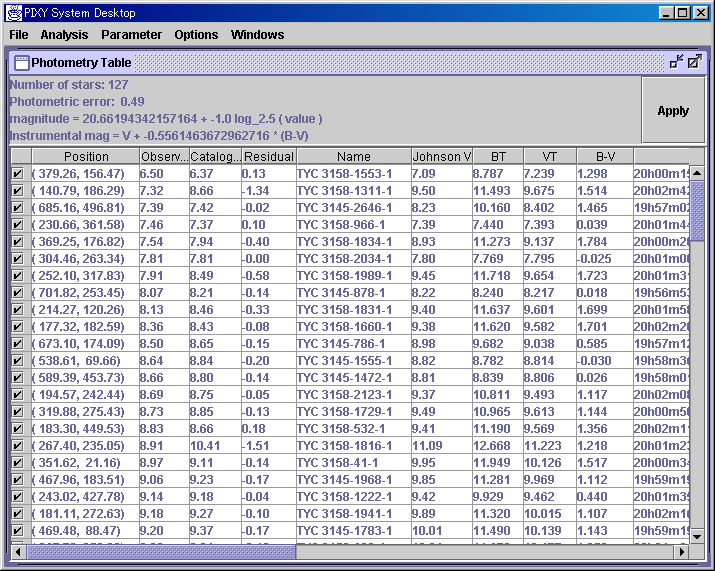# Photometry

 The contents of "Guideline of photometry" are the summary of instructions by Taichi Kato and Seiichiro Kiyota in the VSOLJ(Variable Star Observers' League in Japan) mailing list.

This page introduces how to determine the magnitude of a star properly based on the image examined by the PIXY System 2.

##Guideline of photometry

Depends on the filters, CCD chips and the reference catalogs, there three types of photometry.

### (1) Standard system

When you observe a star using some standard filter, such as V, B, Rc, etc., you have to use catalogs which contains the magnitude in standard system, the same system as the filter you use, as reference. For example, when you observe a star using a standard V filter, you have to refer to a catalog which contains standard V magnitude (Tycho Catalogue, for instance).

When the standard V magnitude of a star is recorded in the catalog as 10.0 mag, the magnitude of another star which is 2.5 times brighter than it will be 9.0 mag.

In principle, standard filters are specific to the CCD chips. You have to use the proper filter for your CCD chip. Instead, the magnitude in the standard system measured as mentioned above is consistent with other observers' data in the same system.

Sometimes the magnitude in some standard system are not recorded in the catalog. Then you can convert from magnitude in other standard systems. For example, when you observe a star using the standard Rc filter, there is no Rc data in the Tycho Catalogue. Then you can convert from V magnitude and B-V value recorded in the catalog into the approximate Rc magnitude following the formula:

Rc = V - 0.5 * (B-V)

and determine the magnitude using this approximate Rc magnitude. When you observe a star using the standard Ic filter, you can convert from B magnitude and B-V value into the approximate Ic magnitude following the formula:

Ic = B - 2.36 * (B-V)
Natali F., Natali G., Pompei E., Pedichini F., 1994, A&A 289, 756
The use of the (B-I) color index and applications of the (B-I) versus (B-V) relationship

### (2) Non-standard system

In case of unfiltered CCD images, or images using non-standard filters (IR-blocking filters, for instance), you have to estimate the feature of your own system at first, then convert from the standard magnitude recorded in the catalog into the specified magnitude for your own system.

In general, the feature of a system is resembled by the following linear equation of standard V magnitude and B-V value:

Instrumental mag = V + k * (B-V)

For some typical CCD chips such as KAF, TC-241, etc., the coefficient of (B-V) is obtained in Arne A. Henden's research:

Henden, A. A., "The M67 Unfiltered Photometry Experiment",
2000, Journal AAVSO vol. 29, page 35.
ftp://ftp.nofs.navy.mil/pub/outgoing/aah/m67/paper/

When you observe a star using other CCD chips, or using non-standard filters, you have to calculate the formula for your own system based on the images at first.

When the formula is obtained, you can convert from the standard V magnitude and B-V value in some catalog (Tycho Catalogue for instance) into the magnitude specified for your own system by the formula, then determine the magnitude based on the converted magnitude.

For example, the standard magnitude of a star are recorded in the catalog as:

V mag = 10.0 mag
B-V = 0.8 mag

If the image is an unfiltered CCD image of KAF chip, the feature of your own system is:

Instrumental mag = V - 0.5174 * (B-V)

by Henden's research. So the magnitude of this star specified for your system will be:

Instrumental mag = 10.0 - 0.5174 * 0.8
= 10.0 - 0.41392
= 9.6

The magnitude of another star which is 2.5 times brighter than it will be 8.6 mag.

The magnitude measured as mentioned above is specified for your CCD chip and filter. It is consistent with other observers' data using the same type of CCD chip and filter. However it is not consistent with other observers' data using difference type of CCD chip or filter.

### (3) Simple magnitude comparison

When the feature of your CCD chip and filter is uncertain, or there are no stars in the image field whose standard magnitude are recorded in the catalog, it is impossible to determine the magnitude in the regular style.

Especially in case of narrow images, only such catalogs as the GSC or the USNO-A2.0 may be available to refer the magnitude.

In those cases, only you can do is to determine the magnitude of a star simply comparing to the magnitude recorded in the catalogs.

For example, the magnitude of a star is recorded in the USNO-A2.0 catalog as:

R mag = 10.0 mag
B mag = 14.0 mag

Then the magnitude of another star which is 2.5 times brighter than it will be: 9.0 mag using the R magnitude, or 13.0 mag using the B magnitude.

The magnitude measured as mentioned above can have a large shift from the true value. Because the base point is not fixed, the magnitude of a star measured from several images shows large scatter. For example, the magnitude of a star can be 10.0 mag in one image, and 11.0 mag in another image.

### (A) Appendix

The (B-V) value of the base star of photometry is 0.0 mag. When there is a star whose (B-V) value is 0.0 mag in the image field, you can determine the true magnitude of any other stars comparing to the brightness of the star, whatever filters you use. In this case, you do not have to obtain the formula for your own system.

However, there are some sorts of stars which cannot be used for photometry even if the (B-V) value is 0.0 mag. So it is not recommended that you determine the magnitude of stars comparing to only one star in the image field, even if the (B-V) value of the star is 0.0 mag.

##Photometry using the PIXY System 2

You have to examine the image as introduced in the Examine Your Image page and save the result in the XML file in advance.

Next you have to identify with the catalog used in photometry as introduced in the Look Up Comets, Asteroids, Variable Stars, Clusters and Nebulae in the Image Field page. For example, if you examine the image using the USNO-A2.0, but would like to determine the magnitude using the Tycho Catalogue considering the feature of your own system, you have to identify with the Tycho Catalogue in advance. After identification, save the result in the XML file. When the catalog used in the examination is also used in the photometry, identification can be passed.

After identification, star data used in photometry are recorded in the XML file. Then let's start the photometry.

First of all, run the PIXY System 2 and select Review in the main menu window.Then a new virtual desktop appears.First, open the XML file. Select the [File] - [Open XML File] menu and open the XML file of the examination result.

Then select the [Analysis] - [Photometry] menu, and a dialog appears to set up the photometry operation.

As introduced in the Guideline of Photometry, there are three types of photometry. When you would like to determine the magnitude in regular style as mentioned in the Standard system or Non-standard system sections, select the Regular Photometry tab. When you would like to determine the magnitude by simple magnitude comparison as mentioned in the Simple magnitude comparison section, select the Simple Comparison tab.

Selecting the Regular Photometry tab, the following window appears.In case of the image taken using the standard filter, check the Standard system and select one filter.

In case of the unfiltered CCD images, look up the combo box. If your CCD chip is in the list, check the Unfiltered CCD image and select the CCD chip.

In case your CCD chip is not in the list, or you use non-standard filters, check the Other instruments. When the feature formula of your system is already obtained, input the coefficient of B-V value. Otherwise, check the Calculate system formula, then the system calculates the feature formula for your system based on the current image, and determines the magnitude using the calculated formula.

Finally, select a reference catalog.

Selecting the Simple Comparison tab, the following window appears.When you check the Average fitting, the system determines the magnitude so that the average of the difference between measured magnitude and catalog magnitude becomes 0.

When you check the Line fitting, the system calculates the linear equation between logarithm of the measured pixel count value and catalog magnitude, then determines the magnitude using the calculated equation.

In general, check the Average fitting.

Finally, select a reference catalog.

After the setting is finished, push the OK button. Then a table appears which shows the magnitude and other data in the catalog, measured magnitude and residuals. On top of the table, the number of data used in photometry, the error of photometry, the equation to convert the pixel count value into magnitude, are output. When you check the Calculate system formula, the feature formula of your own system calculated based on the current image is also output.Selecting the data using the check box in the table, the measured magnitude and the equations output on top of the table are updated. In general, it is recommended to click the Residual column, sort the data in order of residual, and reject illegal data with large residual.In this example, one data with large residual is rejected. The Position value implies that the brightness of this star is wrong because it is at edge. The formula of this example system is calculated as: Instrumental mag = V - 0.6679 * (B-V)

Finally, push the Apply button and the magnitude of all detected stars are determined.

After photometry finished, save the result in the XML file.

After that, click a star on the Detected Stars chart, and you can see the measured magnitude.